# Circuit voltage

Calculate the closed-circuit voltage. Calculate the power on the resistors. R1 = 30 ohms, R2 = 10 ohms. I = 0.1A. U =?. The resistors are connected in series, one after the other.

P1 =  0.3 W
P2 =  0.1 W
U =  4 V

### Step-by-step explanation: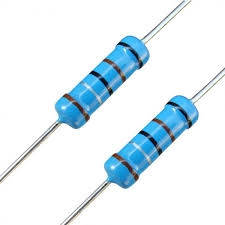Did you find an error or inaccuracy? Feel free to write us. Thank you!## Related math problems and questions:

• Lowest voltage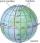Three resistors with resistors R1 = 10 kΩ, R2 = 20 kΩ, R3 = 30 kΩ are connected in series and an external voltage U = 30 V is connected to them. On which resistor is the lowest voltage?
• Two resistorsTwo resistors 20 Ω and 60 Ω are connected in series and an external voltage of 400 V is connected to them. What are the electrical voltages on the respective resistors? Please comment!
• Closed circuitIn a closed circuit, there is a voltage source with U1 = 12 V and with an internal resistance R1 = 0.2 Ω. The external resistance is R2 = 19.8 Ω. Determine the electric current and terminal voltage.
• Resistor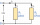Resistor 1 with a resistance of 100 Ohms and resistor 2 with a resistance of 400 Ohms are connected side by side in the circuit. There is a voltage of 80V between the resistor terminals. a) Draw a circuit diagram and write the entered quantities in it b)
• Electric workCalculate the work done by the electric forces passing the current of 0.2 A through the bulb in 10 minutes if the bulb is connected to a 230 V power supply.
• Kirchhoff's lawTwo resistors with 100 Ω and 300 Ω resistors are connected in series. A current of 1.8 A passes through the first 100Ω resistor. What current flows through the second resistor?
• Serial connectionIf the voltage on the first resistor is 6 V and the resistance ratio of the first and second resistors is 1: 3, what will be the voltage across the second resistor if they are connected in series?
• ResistanceA resistor having an electrical resistance of 1.5 k ohms passes an electrical current of 0.1 A. Calculate what voltage is between the terminals of the resistor.
• Electric input powerSolve problems related to electric power: a) U = 120 V, I = 0.5 A, P =? b) P = 200 W, U = 230 V, I =? c) I = 5 A, P = 2200W, U =?
• Direction indicatorAs that which the electrical voltage is connected to the lamp direction indicator 7400 mOhm resistance when current of 1.6 A passes through it?
• Filament of bulbThe filament of bulb has a 1 ohm resistivity and is connected to a voltage 220 V. How much electric charge will pass through the fiber when the electric current passes for 10 seconds?
• The bulbsThe bulbs are connected serially two bulbs and one resistor. Each bulb has a resistivity of 100 ohms and resistor of 60 ohms The voltage source in the circuit is 24 volts. Do you know what voltage to measure on individual appliances?
• Current in the conductorCalculate the current in the conductor (in mA) if it is connected to a 4.5 V voltage source and its resistance is 20 (ohm).
• Resistors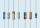Two resistors connected in series give the resulting resistance 245Ω and 60Ω in parallel. Determine the resistance of these resistors.
• Mains voltageHow much electric current flows through the appliance with a resistance of 40kΩ, which is connected to the mains voltage (230 V)?
• Resistance - ohmsIf the resistance of the first resistor is 300 ohms and the resistance of the second resistor is 100 ohms. What is the resulting resistance?
• Two resistors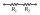Two resistors when they give 25 ohms in series and 4 ohms in parallel what the values of